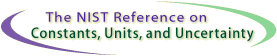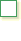SI Unit rules and style conventions
Check List for Reviewing Manuscripts#1General
Only units of the SI and those units recognized for use with the SI are used to express the values of quantities. Equivalent values in other units are given in parentheses following values in acceptable units only when deemed necessary for the intended audience.#2Abbreviations
Abbreviations such as sec, cc, or mps are avoided and only standard unit symbols, prefix symbols, unit names, and prefix names are used.
proper: s or second; cm3 or cubic centimeter; m/s or meter per second
improper: sec; cc; mps#3Plurals
Unit symbols are unaltered in the plural.
proper:
l = 75 cm
improper:
l = 75 cms#4Punctuation
Unit symbols are not followed by a period unless at the end of a sentence.
proper:
The length of the bar is 75 cm.
The bar is 75 cm long.
improper:
The bar is 75 cm. long.#5Multiplication
& division
A space or half-high dot is used to signify the multiplication of units. A solidus (i.e., slash), horizontal line, or negative exponent is used to signify the division of units. The solidus must not be repeated on the same line unless parentheses are used.
proper:
The speed of sound is about 344 m·s-1  (meters per second)
The decay rate of 113Cs is about 21 ms-1  (reciprocal milliseconds)
m/s,  m·s-2,  m·kg/(s3·A),  m·kg·s-3·A-1

m/s,  m s-2,  m kg/(s3 A),  m kg s-3 A-1
improper:
The speed of sound is about 344 ms-1  (reciprocal milliseconds)
The decay rate of 113Cs is about 21 m·s-1  (meters per second)
m ÷ s,  m/s/s,  m·kg/s3/A#6Typeface
Variables and quantity symbols are in italic type. Unit symbols are in roman type. Numbers should generally be written in roman type. These rules apply irrespective of the typeface used in the surrounding text.  For more details, see Typefaces for symbols in scientific manuscripts
proper:
She exclaimed, "That dog weighs 10 kg!"
t = 3 s, where t is time and s is second
T = 22 K, where T is thermodynamic temperature, and K is kelvin
improper:
He exclaimed, "That dog weighs 10 kg!
t = 3 s, where t is time and s is second
T = 22 K, where T is thermodynamic temperature, and K is kelvin#7Typeface
Superscripts and subscripts are in italic type if they represent variables, quantities, or running numbers. They are in roman type if they are descriptive.
 subscript categorytypefaceproper usage quantity italic cp, specific heat capacity at constant pressure descriptive roman mp, mass of a proton running number italic#8Abbreviations
The combinations of letters "ppm," "ppb," and "ppt," and the terms part per million, part per billion, and part per trillion, and the like, are not used to express the values of quantities.
proper: 2.0 µL/L; 2.0 x 10-6 V;
4.3 nm/m; 4.3 x 10-9 l;
7 ps/s; 7 x 10-12 t,
where V, l, and t are the quantity symbols for volume, length, and time.
improper: "ppm," "ppb," and "ppt," and the terms part per million, part per billion, and part per trillion, and the like#9Unit
modifications
Unit symbols (or names) are not modified by the addition of subscripts or other information. The following forms, for example, are used instead.
proper: Vmax = 1000 V
a mass fraction of 10 %
improper: V= 1000 Vmax
10 % (m/m) or 10 % (by weight)#10Percent The symbol % is used to represent simply the number 0.01. proper: l1 = l2(1 + 0.2 %), or D = 0.2 %, where D is defined by the relation D = (l1 - l2)/l2. improper: the length l1 exceeds the length l2 by 0.2 %#11Information & units Information is not mixed with unit symbols or names. proper: the water content is 20 mL/kg improper: 20 mL H2O/ kg 20 mL of water/ kg#12Math notation It is clear to which unit symbol a numerical value belongs and which mathematical operation applies to the value of a quantity. proper: 35 cm x 48 cm 1 MHz to 10 MHz or (1 to 10) MHz 20 °C to 30 °C or (20 to 30) °C 123 g ± 2 g or (123 ± 2) g 70 % ± 5 % or (70 ± 5) % 240 x (1 ± 10 %) V improper: 35 x 48 cm 1 MHz-10 MHz or 1 to 10 MHz 20 °C-30 °C or 20 to 30 °C 123 ± 2 g 70 ± 5 % 240 V ± 10 % (one cannot add 240 V and 10 %)#13Unit symbols & names Unit symbols and unit names are not mixed and mathematical operations are not applied to unit names. proper: kg/m3, kg · m-3, or kilogram per cubic meter improper: kilogram/m3, kg/cubic meter, kilogram/cubic meter, kg per m3, or kilogram per meter3.#14Numerals & unitsymbols Values of quantities are expressed in acceptable units using Arabic numerals and symbols for units. proper: m = 5 kg the current was 15 A improper: m = five kilograms m = five kg the current was 15 amperes#15Unit spacing There is a space between the numerical value and unit symbol, even when the value is used in an adjectival sense, except in the case of superscript units for plane angle. proper: a 25 kg sphere an angle of 2° 3'  4" If the spelled-out name of a unit is used, the normal rules of English apply: "a roll of 35-millimeter film." improper: a 25-kg sphere an angle of 2 ° 3 ' 4 "#16Digit spacing The digits of numerical values having more than four digits on either side of the decimal marker are separated into groups of three using a thin, fixed space counting from both the left and right of the decimal marker. Commas are not used to separate digits into groups of three. proper: 15 739.012 53 improper: 15739.0125315,739.012 53#17Quantity equations Equations between quantities are used in preference to equations between numerical values, and symbols representing numerical values are different from symbols representing the corresponding quantities. When a numerical-value equation is used, it is properly written and the corresponding quantity equation is given where possible. proper: (l/m) = 3.6-1 [v/(km/h)](t/s) improper: l = 3.6-1 vt, accompanied by text saying, "where l is in meters, v is in kilometers per hour, and t is in seconds"#18Standardsymbols Standardized quantity symbols are used. Similarly, standardized mathematical signs and symbols are used. More specifically, the base of "log" in equations is specified when required by writing loga x (meaning log to the base aof x), lb x (meaning log2 x), ln x (meaning loge x), or lg x (meaning log10 x). proper: tan x R for resistance Ar for relative atomic mass improper: tg x for tangent of x words, acronyms, or ad hoc groups of letters#19Weight vs. mass When the word "weight" is used, the intended meaning is clear. (In science and technology, weight is a force, for which the SI unit is the newton; in commerce and everyday use, weight is usually a synonym for mass, for which the SI unit is the kilogram.)#20Quotientquantity A quotient quantity is written explicitly. proper: mass divided by volume improper: mass per unit volume#21Object & quantity An object and any quantity describing the object are distinguished. (Note the difference between "surface" and "area," "body" and "mass," "resistor" and "resistance," "coil" and "inductance.") proper: A body of mass 5 g improper: A mass of 5 g#22Obsolete Terms The obsolete terms normality, molarity, and molal and their symbols N, M, and m are not used. proper: amount-of-substance concentration of B (more commonly called concentration of B), and its symbol cB and SI unit mol/m3 (or a related acceptable unit) molality of solute B, and its symbol bB or mB and SI unit mol/kg (or a related unit of the SI) improper: normality and the symbol N, molarity and the symbol M molal and the symbol m